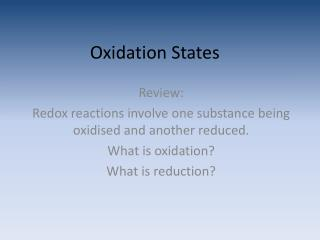DownloadDownload PresentationOxidation States

# Oxidation States

Télécharger la présentation## Oxidation States

- - - - - - - - - - - - - - - - - - - - - - - - - - - E N D - - - - - - - - - - - - - - - - - - - - - - - - - - -
##### Presentation Transcript

1. Oxidation States Review: Redox reactions involve one substance being oxidised and another reduced. What is oxidation? What is reduction?

2. Review: • Oxidation always involves loss of e-; it can also involve the gain of oxygen and/or loss of hydrogen • Reduction always involves gain of e-; it can also involve loss of oxygen and/or gain of hydrogen.

3. The oxidation state describes the extent to which an element has been oxidised or reduced. • The oxidation state can be identified by oxidation numbers. • Oxidation numbers can be seen as a method to account for electrons taking part in a reaction.

4. Oxidation numbers can be used to establish what element has been oxidised and what element has been reduced in a reaction. • There is an increase in oxidation number with oxidation • A decrease in oxidation number with reduction • Oxidation numbers are assigned to elements.

5. www.youtube.com/watch?v=EHe8-AFMsMAShort Video clip on the Oxidation Number trends in the Periodic Table.

6. Oxidation number rules

7. Examples: • Calculate the ON of nitrogen in the following compounds NO2- O = -2 x 2 = -4 Charge = -1 N = +3

8. NH3

9. N2O

10. NO

11. HNO3

12. Review: • An increase in oxidation number is… • A decrease in oxidation number is…

13. Recognising oxidation & reduction PbO2(s) + C(s) -> Pb(s) + CO2(g) ?,2(-2) 0 0 ?,2(-2) 4,2(-2) 0 0 4,2(-2) Carbon oxidised(ON increased from 0 to 4) Lead oxide reduced(ON reduced from 4 to 0)

14. Mg(s) + 2H+ -> Mg2+(aq) + H2(g) 0 2(+1) +2 0 Magnesium oxidised (ON, increased 0 to 2) Hydrogen ions reduced (ON, decreased +1 to 0)

15. H+(aq) + OH-(aq) -> H2O(l) Assign oxidation numbers +1 (-2+1) (2+1)(-2) no change in oxidation number .. Not redox

16. Mn2+ MnO2 MnO4- S2Cl2 SCl2 SO2 SO3 S2O32- Cr2O72- Oxidation number quick check. Calculate the oxidation number of the bold element +2 +4 +7 +1 +2 +4 +6 +2 +6

17. Work out the Oxidation state of each atom • MnO4- Mn2+ • Cr2072-  Cr3+ • Cl2  Cl- • H2O2  H2O • MnO2  Mn2+ • NO3-  NO2 • NO3-  NO

18. Oxidants and Reductants • An oxidant is defined as an electron acceptor. • An oxidant can also be called an oxidising agent. • An oxidant is therefore reduced. • A reductant is defined as an electron donor. • A reductant can also be called a reducing agent. • A reductant is therefore oxidised.

19. Review: • What is the oxidation number of: a.) Manganese in manganese dioxide, MnO2. b.) Nitrogen in nitrate ions, NO3- • Give the oxidation number of each type of atom present in the following: a.) Mg b.) SO2 c.) MgO d.) S8 e.) Ag+ f.)NaHCO3 g.)H2SiO3 • Element M has O.N +3. The most likely formula for the oxide of M is: a.) MO b.) M2O c.) M2O3 d.) MO2

20. Recall… • The reactant which is oxidised is called the reductant (or reducing agent) since it reduces the other reactant. • The reactant which is reduced is called the oxidant (or oxidising agent) since it oxidises the other reactant. e.g. 2Mg(s) + O2(g) 2MgO(s) Mg is the because . Oxygen is the because .

21. Oxidation in terms of oxygen transfer Oxidation is the gain of oxygen, reduction is the loss of oxygen Example: 2Fe2O3(s) + 3C(s) 4Fe(s) + 3CO2(g) oxygen transfer • Fe2O3 is reduced since it loses oxygen • C is oxidised since it gains oxygen to form CO2 • Fe2O3 is the oxidant and C is the reductant.

22. Oxidation in terms of hydrogen transfer Oxidation is the loss of hydrogen, reduction is the gain of hydrogen Example: 2H2S(g) + O2(g) 2S(s) + 2H2O(l) Hydrogen transfer H2S is oxidised because H is lost O2 is reduced because H is gained to form H2O H2S is the reductant and O2 is the oxidant.

23. Oxidation in terms of electron transfer Oxidation is loss of electrons, reduction is gain of electrons Example: Zn(s) + Cu2+(aq) Zn2+(aq) + Cu(s) 2e- Zn metal loses electrons and is oxidised. Copper ions gain electrons to form copper metal Zinc is the reductant and copper ions are the oxidant.

24. Questions • For the reaction 2PbO + C 2Pb + CO2 a.) Use the transfer of oxygen definition of redox to identify the oxidant and reductant. b.) Use oxidation numbers to show the same oxidant and reductant would be identified. Explain your answers.

25. Questions 2. For the reaction Fe + Cu2+ Fe2+ + CO2 a.) Use transfer of electrons to identify the oxidant and reductant b.) Show that, using oxidation numbers, the same oxidant and reductant would be identified.